# Double square well modelThis article is a 'stub' page, it has no, or next to no, content. It is here at the moment to help form part of the structure of SklogWiki. If you add sufficient material to this article then please remove the {{Stub-general}} template from this page.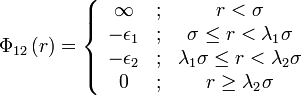$\Phi_{12}\left( r \right) = \left\{ \begin{array}{ccc} \infty & ; & r < \sigma \\ - \epsilon_1 & ; &\sigma \le r < \lambda_1 \sigma \\ - \epsilon_2 & ; &\lambda_1\sigma \le r < \lambda_2 \sigma \\ 0 & ; & r \ge \lambda_2 \sigma \end{array} \right.$
where$\Phi_{12}(r)$ is the intermolecular pair potential,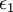$\epsilon_1$ and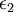$\epsilon_2$ are the well depths (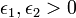$\epsilon_1,\epsilon_2>0$),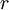$r$ is the distance between site 1 and site 2 where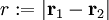$r := |\mathbf{r}_1 - \mathbf{r}_2|$, σ is the hard core diameter and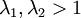$\lambda_1,\lambda_2>1$